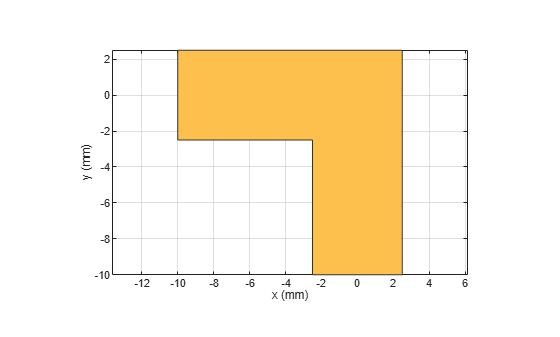# bendRightAngle

Create right-angle bend shape on X-Y plane

Since R2021b

## Description

Use the `bendRightAngle` object to create a right-angle bend shape on the X-Y plane.

Note

This shape object supports behavioral modeling. For more information, see Behavioral Models.

## Creation

### Syntax

``bend = bendRightAngle``
``bend = bendRightAngle(Name=Value)``

### Description

example

````bend = bendRightAngle` creates a right-angle bend shape on the X-Y plane.```

example

````bend = bendRightAngle(Name=Value)` sets Properties using one or more name-value arguments. For example, ```bendRightAngle(ReferencePoint=[1 1])``` creates a right-angle bend shape with the reference point at ```[1 1]```. Properties not specified retain their default values.```

## Properties

expand all

Name of the right-angle bend shape, specified as a character vector or a string scalar.

Example: `bend = bendRightAngle(Name="bendrightangle1")`

Data Types: `char`

Reference point for the right-angle bend shape in Cartesian coordinates, specified as a two-element vector.

Example: `bend = bendRightAngle(ReferencePoint=[1 2])`

Data Types: `double`

Length of the right-angle bend shape in meters, specified as a two-element vector.

Example: `bend = bendRightAngle(Length=[0.0500 0.0500])`

Data Types: `double`

Width of the right-angle bend shape in meters, specified as a two-element vector.

Example: `bend = bendRightAngle(Width=[0.0200 0.0200])`

Data Types: `double`

## Object Functions

 `add` Boolean unite operation on two RF PCB shapes `and` Shape1 & Shape2 for RF PCB shapes `area` Calculate area of RF PCB shape in square meters `intersect` Boolean intersection operation on two RF PCB shapes `mesh` Change and view mesh properties of metal or dielectric in PCB component `minus` Shape1 - Shape2 for RF PCB shapes `plus` Shape1 + Shape2 for RF PCB shapes `rotate` Rotate RF PCB shape about defined axis `rotateX` Rotate RF PCB shape about x-axis `rotateY` Rotate RF PCB shape about y-axis and angle `rotateZ` Rotate RF PCB shape about z-axis `subtract` Boolean subtraction operation on two RF PCB shapes `scale` Change size of RF PCB shape by fixed amount `show` Display PCB component structure or PCB shape `translate` Move RF PCB shape to new location

## Examples

collapse all

Create a right-angle bend with default properties.

`bend = bendRightAngle`
```bend = bendRightAngle with properties: Name: 'myRightAnglebend' ReferencePoint: [0 0] Length: [0.0100 0.0100] Width: [0.0050 0.0050] ```

View the shape.

`show(bend)`Create a right-angle bend shape of lengths of 10 m and 2 m, width of 2m, and rotate it about the Z-axis by 60 degrees.

```bend = bendRightAngle(Length=[10 2],Width=[2 2]); bend = rotateZ(bend,60)```
```bend = bendRightAngle with properties: Name: 'myRightAnglebend' ReferencePoint: [0 0] Length: [10 2] Width: [2 2] ```
`show(bend)`Mesh the right-angle bend shape at a maximum edge length of 1 m.

`mesh(bend,MaxEdgeLength=1)`## Version History

Introduced in R2021b